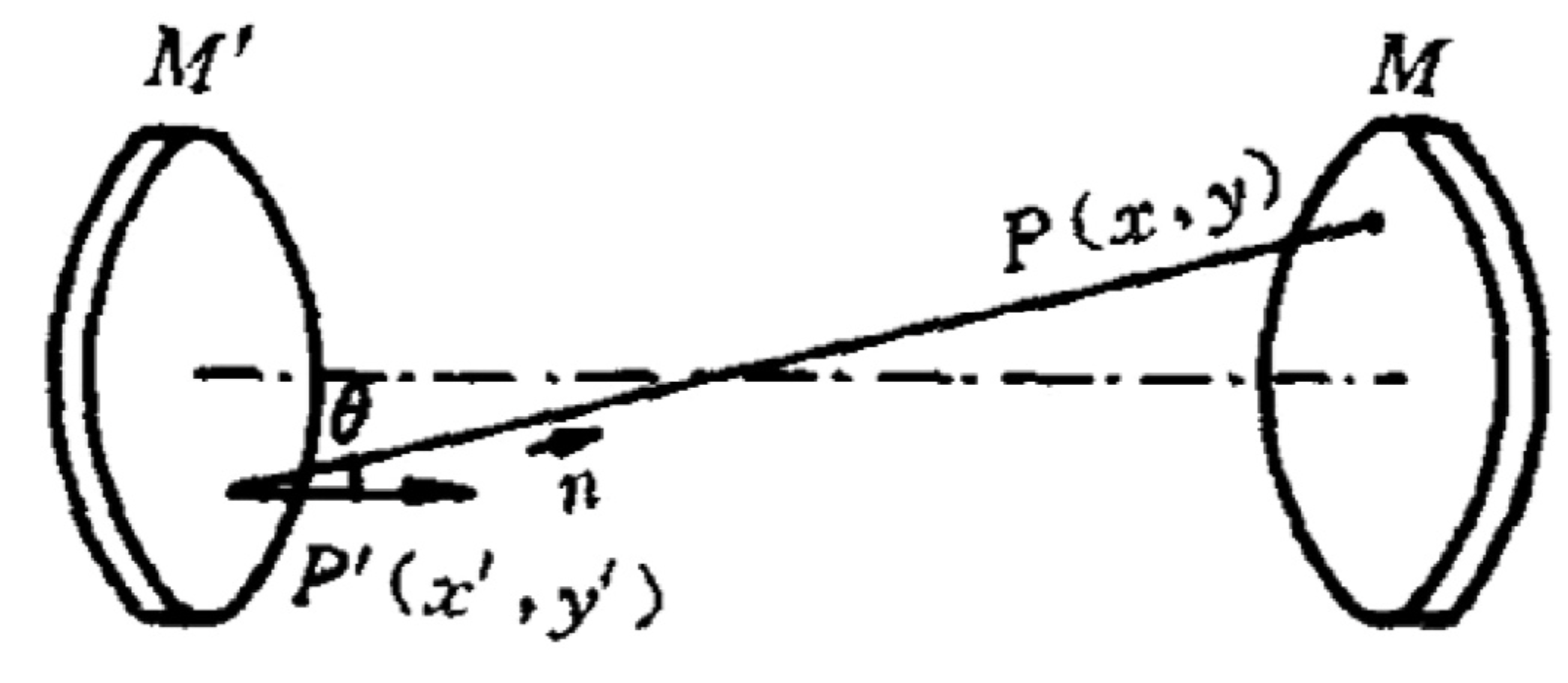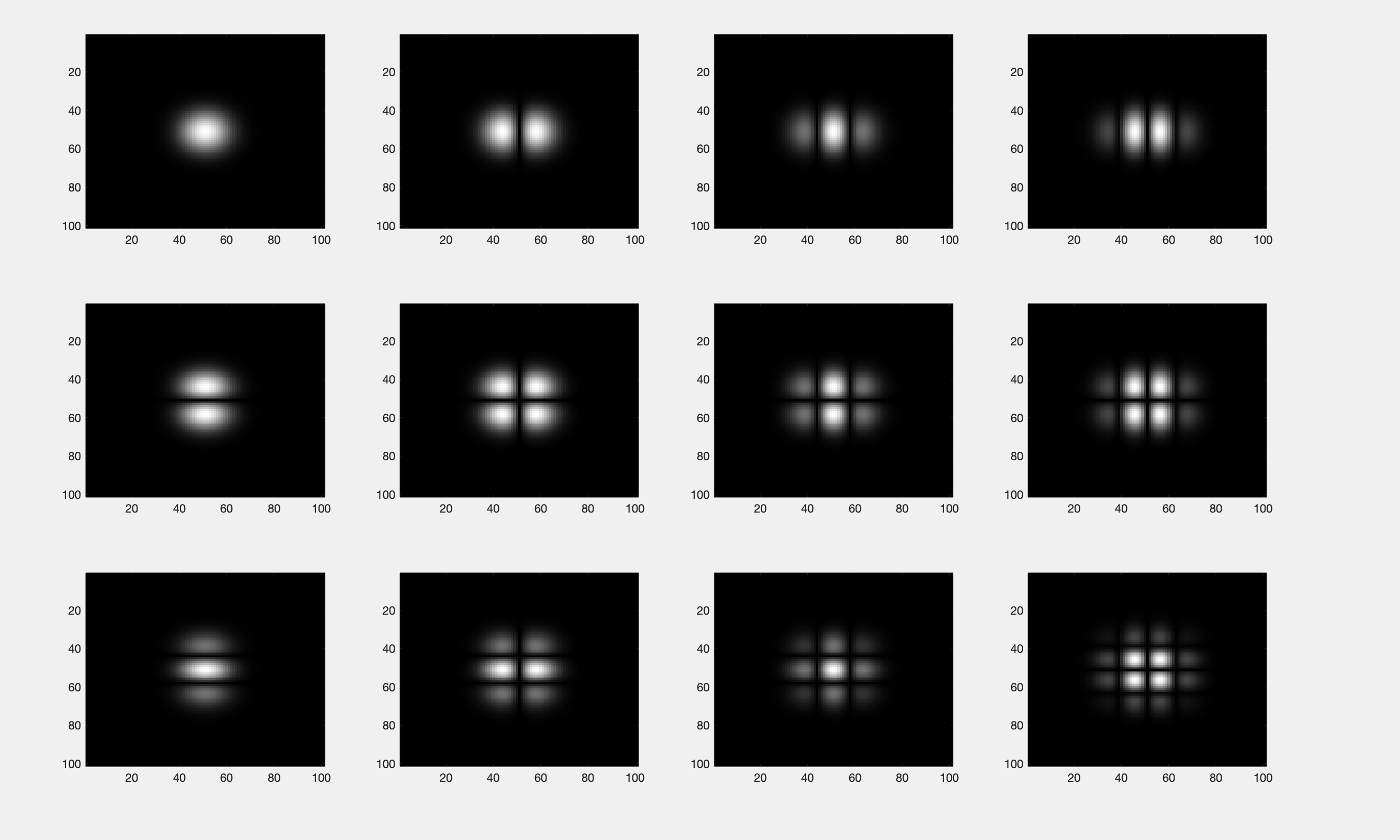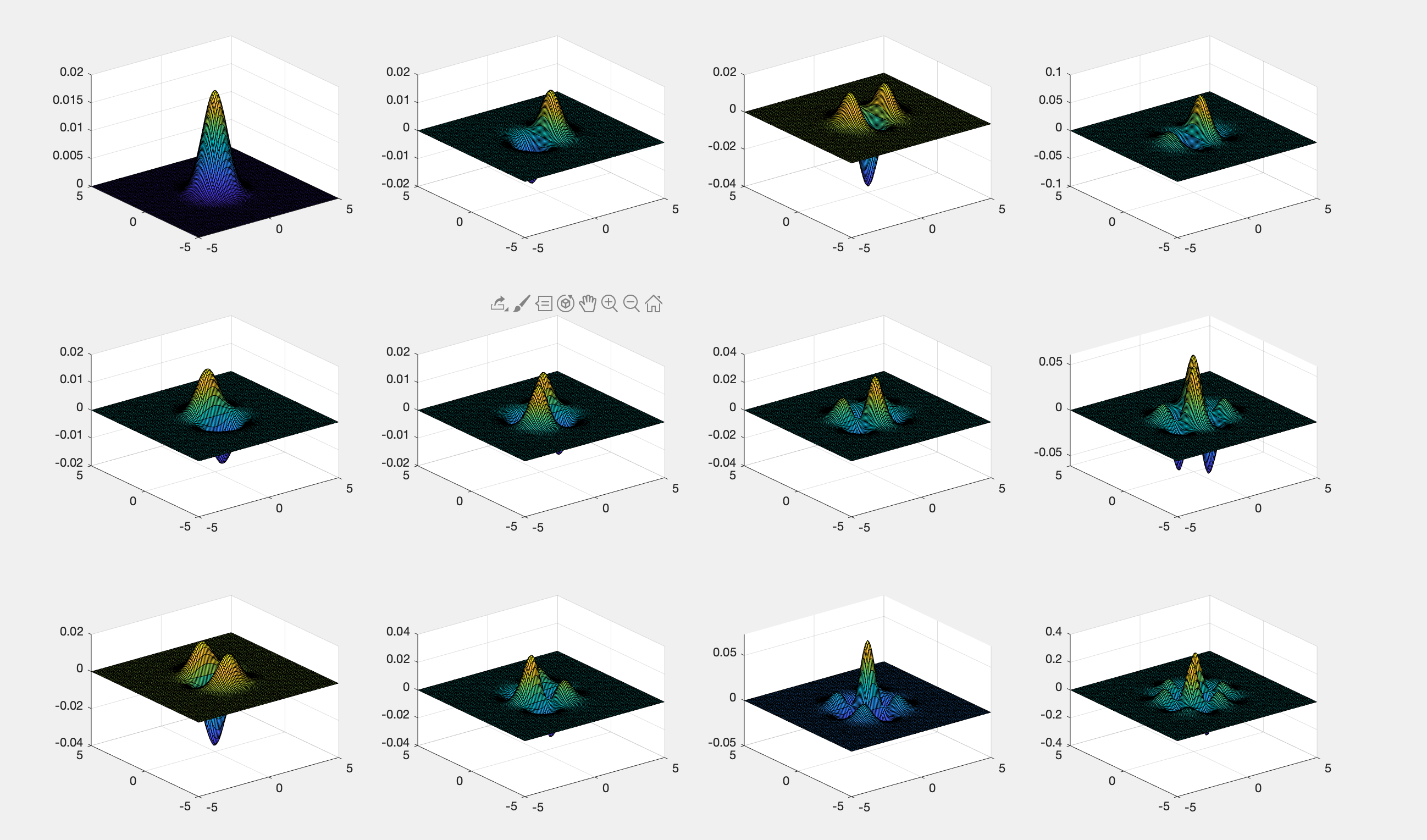• 几个公式代表了不同条件下的高斯光束光强分布 Hm(x)H_m(x)Hm​(x) F=e−x2+y2w2F = e^{-\frac{x^2+y^2}{w^2}}F=e−w2x2+y2​ Hm2(x)F2H_m^{2}(x)F^2Hm2​(x)F2 2 MATLAB实现 clear clc %% 初始值 x = -2*pi:pi/...
目录1 物理模型2 MATLAB实现2.1 光斑效果展示2.2 高斯光束光强分布的三维模型2.3 代码实现3 知识拓展3.1 高斯分布是什么？3.2 单独实现高阶高斯分布
1 物理模型
在激光器的方形镜对称共焦腔中，厄米特—高斯分布描述了腔镜上自再现模的场分布，对其进行数值模拟，可以观察不同阶数下本征模的场分布情况(x，y)表示镜面上的任意点坐标，腔长：L，光波波长：λ，原点选在镜面中心，则在近轴近似条件下 L,R>>α>>λ ，腔镜镜面上的电场复振幅可以表示为
$u_{mn} \approx C_{mn}H_m(X)Hn(Y)e^{\frac{X^2+Y^2}{2}}$
其中，m=0, 1, 2, …和 n=0, 1, 2…，m、n 为横截面上 x、y 方向出现的节点；$C_{mn}$为与 m、n 有关的常量Hm(X)、Hn(Y)为厄密多项式，可以表示为
$H_m(X) =(-1)^me^{X^2}\frac{d^m(e^{-X^2})}{dX^m}$
$H_0(X) = 1,H_1(X) = 2X,H_2(X)=4X^2-2,H_3(X)=-8x^3+12x$
也可以写为
$u_{mn}\approx C_{mn}F_m(X)F(Y)$
$F_(X) = H_m(X)e^{-X^2/2}$
$F_(Y) = H_n(X)e^{-Y^2/2}$
因此不同阶数的光场分布对应光斑分布可以表示为：激光的模式常用微波中的表示方法，即$TEM_{mn}$ ，其中$TEM_{00}$是基模，可以看出 m 和 n 的值越大，光场也越向外扩展。当 m=0，n=0 时，光场分布为基模高斯光束$TEM_{00}$，其传播场的振幅分布可写为
$U_{00} = exp(-\frac{2}{1+\zeta ^2} \frac{x^2+y^2}{w_s^2})$
$\zeta = 2z/L,w_s = \sqrt{\lambda L/\pi}$
$z为传播距离，L为腔长，\lambda 为波长$
其零点是对称共焦腔的中心。高斯光束的传播呈现双曲线型的形态，波长一定情况下，共焦腔内 z 处的截面半径大小仅取决于腔长 L。高斯光束的束腰半径可以写为
$w_0 = \sqrt{\frac{\lambda L}{2\pi}}= \frac{1}{\sqrt{2}}w_s$
2 MATLAB实现
2.1 光斑效果展示2.2 高斯光束光强分布的三维模型2.3 代码实现
clear all
close all

x=[-5:0.1:5].*1e-3;            % 坐标范围
lambda=632.8e-9;               % 波长
L=10;                          % 腔长
X=x.*sqrt(2*pi./(lambda.*L));
[X1,Y1]=meshgrid(X,X);         % X和Y的坐标矩阵

%% 厄米特函数
% H0-H3
H0X=1;
H0Y=1;
H1X=2.*X1;
H1Y=2.*Y1;
H2X=4.*X1.^2-2;
H2Y=4.*Y1.^2-2;
H3X=-8.*X1.^3+12.*X1;
H3Y=-8.*Y1.^3+12.*Y1;

%% 厄米特——高斯函数
% F0-F3
FX0=H0X.*exp(-X1.^2-2);
FY0=H0Y.*exp(-Y1.^2-2);
FX1=H1X.*exp(-X1.^2-2);
FY1=H1Y.*exp(-Y1.^2-2);
FX2=H2X.*exp(-X1.^2-2);
FY2=H2Y.*exp(-Y1.^2-2);
FX3=H3X.*exp(-X1.^2-2);
FY3=H3Y.*exp(-Y1.^2-2);

%% 振幅分布

u00=FX0.*FY0;         % TEM00
subplot(3,4,1)
imagesc(abs(u00))

u10=FX1.*FY0;         %  TEM10
subplot(3,4,2)
imagesc(abs(u10))

u20=FX2.*FY0;         % TEM20
subplot(3,4,3)
imagesc(abs(u20))

u30=FX3.*FY0;         % TEM30
subplot(3,4,4)
imagesc(abs(u30))

u01=FX0.*FY1;         % TEM01
subplot(3,4,5)
imagesc(abs(u01))

u11=FX1.*FY1;         % TEM11
subplot(3,4,6)
imagesc(abs(u11))

u21=FX2.*FY1;         % TEM21
subplot(3,4,7)
imagesc(abs(u21))

u31=FX3.*FY1;         % TEM31
subplot(3,4,8)
imagesc(abs(u31))

u02=FX0.*FY2;         % TEM02
subplot(3,4,9)
imagesc(abs(u02))

u12=FX1.*FY2;         % TEM12
subplot(3,4,10)
imagesc(abs(u12))

u22=FX2.*FY2;         % TEM22
subplot(3,4,11)
imagesc(abs(u22))

u33=FX3.*FY3;         % TEM33
subplot(3,4,12)
imagesc(abs(u33))

colormap gray;         %显示灰度图

3 知识拓展
3.1 高斯分布是什么？说白了就是上图中右下角的山坡型的图形。这个山坡型就是下面三维高斯光束的横截面。所以高斯光束就和高斯分布结合起来了。这时候，我将三种图对应起来，有没有发现共通点???从左开始的第一张是高斯分布图，中间张是高斯光束光强三维分布图，最右边是高斯光束光强从三维图的顶部角度看上去的光斑。所以在下面要想实现光斑，只需要画出高斯分布的三维图形，从顶部角度视角展示图形就能得到光斑。
附上绘制这张图的代码
clear all
close all

x=[-5:0.1:5].*1e-3;            % 设定腔镜坐标
lambda=632.8e-9;               % 设定激光器出光频率
L=10;                           % 设定激光腔腔长
X=x.*sqrt(2*pi./(lambda.*L));
Y= X
[X1,Y1]=meshgrid(X,X);         % 获取X和Y的坐标矩阵

% 设定厄米特函数，n和m只从0取到2 %
% 绘制三维的
H0X=1;
H1X=2.*X1;
H2X=4.*X1.^2-2;
H0Y=1;
H1Y=2.*Y1;
H2Y=4.*Y1.^2-2;

% 三维公式
FX0=H0X.*exp(-X1.^2-2);  % 生成厄米特——高斯函数
FX1=H1X.*exp(-X1.^2-2);
FX2=H2X.*exp(-X1.^2-2);

FY0=H0Y.*exp(-Y1.^2-2);
FY1=H1Y.*exp(-Y1.^2-2);
FY2=H2Y.*exp(-Y1.^2-2);

% 二维公式
H0X1=1;
H1X1=2.*X;
H2X1=4.*X.^2-2;
H0Y1=1;
H1Y1=2.*Y;
H2Y1=4.*Y.^2-2;

F0X=H0X1.*exp(-X.^2-2);  % 生成厄米特——高斯函数
F1X=H1X1.*exp(-X.^2-2);
F2X=H2X1.*exp(-X.^2-2);

F0Y=H0Y1.*exp(-Y.^2-2);
F1Y=H1Y1.*exp(-Y.^2-2);
F2Y=H2Y1.*exp(-Y.^2-2);

% 00
I2=FX0.^2.*FY0.^2;         % 考虑X和Y两个方向分布
I1=F0X.^2.*F0Y.^2;
subplot(3,3,1)
plot(X,I1)
subplot(3,3,2)
surf(X1,Y1,I2)
subplot(3,3,3)
surf(X1,Y1,I2)
view(2)

% 01
I2=FX0.^2.*FY1.^2;         % 考虑X和Y两个方向分布
I1=F0X.^2.*F1Y.^2;
subplot(3,3,4)
plot(X,I1)
subplot(3,3,5)
surf(X1,Y1,I2)
subplot(3,3,6)
surf(X1,Y1,I2)
view(2)

% 02
I2=FX0.^2.*FY2.^2;         % 考虑X和Y两个方向分布
I1=F0X.^2.*F2Y.^2;
subplot(3,3,7)
plot(X,I1)
subplot(3,3,8)
surf(X1,Y1,I2)
subplot(3,3,9)
surf(X1,Y1,I2)
view(2)

3.2 单独实现高阶高斯分布其中用到的高斯分布的公式
$H_m(x)$
$F = e^{-\frac{x^2+y^2}{w^2}}$
$H_m^{2}(x)F^2$
clear
clc

%% 初始值
x = -2*pi:pi/100:2*pi;% 横坐标范围
w = 2 % F 函数中w取值
yy =x % 纵坐标范围

%% 画光强分布
figure(2)          % define figure
% 【1 1】个图
subplot(3,4,1);
plot([-10,10],[10,10],'linewidth',2)

% 【1 2】个图
subplot(3,4,2);
y = fx(x,yy,w)
plot(x,y,'linewidth',2);
% 【1 3】个图
subplot(3,4,3);     % subplot(x,y,n)x表示显示的行数，y表示列数，n表示第几幅图片
y = hf(x,yy,w,hx1(x),fx(x,yy,w))
plot(x,y,'linewidth',2);

% 【1 4】个图
subplot(3,4,4);
y = hf2(x,yy,w,hx1(x),fx(x,yy,w))
plot(x,y,'linewidth',2);

% 【2 1】个图
% 画45度斜线
subplot(3,4,5);
y = hx2(x)
plot(x,y,'linewidth',2);

% 【2 2】个图
subplot(3,4,6);
y = fx(x,yy,w)
plot(x,y,'linewidth',2);

% 【2 3】个图
subplot(3,4,7);
y = hf(x,yy,w,hx2(x),fx(x,yy,w))
plot(x,y,'linewidth',2);

% 【2 4】个图
subplot(3,4,8);
y = hf2(x,yy,w,hx2(x),fx(x,yy,w))
plot(x,y,'linewidth',2);

% 【4 1】个图
% 画y=x^2-10
subplot(3,4,9);
y = hx3(x)
plot(x,y,'linewidth',2);

% 【4 2】个图
subplot(3,4,10);
y = fx(x,yy,w)
plot(x,y,'linewidth',2);

% 【4 3】个图
subplot(3,4,11);
y = hf(x,yy,w,hx3(x),fx(x,yy,w))
plot(x,y,'linewidth',2);

% 【4 4】个图
subplot(3,4,12);
y = hf2(x,yy,w,hx3(x),fx(x,yy,w))
plot(x,y,'linewidth',2);

%% 函数
% 绘制水平线函数
function r=hx1(x)
r =1
end
% 绘制45度直线函数
function r=hx2(x)
r =2.*x
end

% 绘制凹曲线函数
function r=hx3(x)
r =4.*x.^2-2
end

% 绘制F的函数
function r =fx(x,y,w)
r = exp(-(x.*2+y.^2)./(w^2))
end

% 绘制Hm(x)*F
function r =hf(x,y,w,source,fun)
r = fun.*source
end
% 绘制Hm(x)^2*F^2的函数
function r =hf2(x,y,w,source,fun)
r = (fun.^2).*(source.^2)
end

对于绘制出来的图形，并没有理想中的那么均匀，但是趋势是大致相同的，有两个原因
（1）原始教材中的图形，是理想化的图像，只是为了突出表述了每个图形的特点。
（2）本人绘制的图形，选择的参数只是随机选择的，比如F函数中w和y的取值仅仅是随机取的。如果想要实现最理想化的图形，需要慢慢的调参


展开全文MATLAB
• 蒋跃文【摘要】根据高斯光束光强分布公式,讨论了P(x)%/(1-P(x)%)为任意值时,高斯光束光斑半径与刀口-光斑中心距比值及相应的光斑半径的测量误差;根据理论分析结果,在P(x)%为不同值条件下,对基模高斯光束光斑半径进行...
刀口法测量高斯光束光斑半径研究杨晓冬;邵建新;廖生鸿;谭锦业;周杰;蒋跃文【摘要】根据高斯光束光强分布公式,讨论了P(x)%/(1-P(x)%)为任意值时,高斯光束光斑半径与刀口-光斑中心距比值及相应的光斑半径的测量误差;根据理论分析结果,在P(x)%为不同值条件下,对基模高斯光束光斑半径进行了实验测量,实验结果与理论分析符合较好.【期刊名称】《激光与红外》【年(卷),期】2009(039)008【总页数】4页(P829-832)【关键词】刀口法;高斯光束光斑半径;透光百分比【作者】杨晓冬;邵建新;廖生鸿;谭锦业;周杰;蒋跃文【作者单位】广东梅州嘉应学院物理学院,广东,梅州,514015;新疆石河子大学物理系,新疆,石河子,832003;广东梅州嘉应学院物理学院,广东,梅州,514015;广东梅州嘉应学院物理学院,广东,梅州,514015;广东梅州嘉应学院物理学院,广东,梅州,514015;广东梅州嘉应学院物理学院,广东,梅州,514015【正文语种】中文【中图分类】工业技术第39卷第8期2009年8月激光与红外LASER＆INFRAREDVol.39 , No.8August,2009文章编号：1001-5078f2009)08-0829 -04·激光技术与应用刀
展开全文• 根据ABCD传输矩阵和Collins衍射积分公式,通过光束坐标与腔体坐标间的转换关系,推导高斯平顶光束经过透镜后,激光间接驱动聚变装置腔内不同角度横截面的光场分布解析表达式,着重分析了光束入射角对焦点处及腔内光强...
• 基于分数傅里叶变换(FRFT)与维格纳分布函数(WDF)旋转等效的性质,推导出了双曲余弦-高斯(ChG)光束在分数傅里叶变换面上光强分布和束宽的解析公式,研究了双曲余弦-高斯光束光强和束宽随分数傅里叶变换阶数的变化规律,...
• 研究表明，左手材料对双曲正弦平方高斯光束轴上光强和横向光强分布规律的影响是使光强呈对称分布；材料的负折射率会影响光束轴上光强极大值的位置；轴上光强和横向光强均随传输距离和偏心参数的改变而变化，即双曲...
• 基于该模型,通过计算大量光子经光学系统的传播轨迹可获得高斯光束的几何构形、光强分布、光子光程分布及其传播方向。对实际非球面光学系统的计算结果充分验证了该方法可全面、准确地模拟高斯光束的传输特性,且数值...
• 在空心高斯光束模型的基础上,由初始光场的光强分布,利用柱坐标下的柯林斯公式,导出了空心锥状双高斯光束经过傍轴光学系统的解析传输变换式由传输变换式计算分析了空心锥状双高斯光束在自由空间的可控传输特性,及在1,...
• 建立了线阵高斯光束的相干合成数学模型，利用Collins衍射积分公式以及将硬边光阑窗口函数分解为有限个复高斯函数之和的方法，推导了相干合成线阵高斯光束通过猫眼目标和朗伯漫反射体反射后的解析光强分布公式。...
• 研究表明，厄米-拉盖尔-高斯光束在分数傅里叶变换面上的光强分布受参量α,模指数m,n和分数傅里叶变换阶数p的影响。光强分布随p和α周期性变化，周期分别为2,2π。此外，厄米-拉盖尔-高斯光束通过分数傅里叶变换系统...
• 基于广义惠更斯-菲涅耳衍射积分公式，推导了双曲正弦-高斯光束通过像散透镜聚焦的场分布解析表达式，并用数值计算研究了双曲正弦-高斯光束在像散透镜焦平面上或焦平面附近的光强分布与相位特性。理论分析与数值计算...
• 在此基础上,进一步研究了空间滤波器具有像传递功能时,高斯光束和平顶高斯光束(N=12)通过空间滤波器的传输特性,得出了截断参数与滤波后光强分布的关系,并证实了此方法也可用于计算其他类型光束通过空间滤波器的传输。
• 利用物理光学的相关知识、Collins衍射积分公式以及硬边光阑的复高斯函数分解法, 推导得到相干基模高斯光束通过卡塞格伦镜头原路返回点处的反射光时间分布的解析表达式。数值仿真分析了条纹间距和中心遮挡比对卡塞...
• 利用Richards-Wolf矢量衍射公式,获得圆偏振涡旋贝塞尔-高斯光束经具有初级慧差高数值孔径系统聚焦后的三维光场复振幅函数,模拟了不同慧差系数下圆偏振涡旋贝塞尔-高斯光束的聚焦光场。研究表明,初级慧差使圆偏振涡旋...
• 采用广义惠更斯-菲涅耳衍射积分公式推导出厄米-余弦-高斯光束...光束参数不仅会改变厄米-余弦-高斯光束的轴上光强分布，还会改变横向光强分布，但对厄米-余弦-高斯光束轴上最大光强和横向最大光强的位置均没有影响。
• 基于柯林斯公式，推导出余弦高斯光束在有球差和无球差的分数傅里叶变换系统中的场分布，并以Lohmann Ⅰ型系统为例，进行数值模拟计算，研究了余弦高斯光束通过有球差和无球差的分数傅里叶变换系统后输出面的横向光强...
• 提出了用多束厄米-高斯光束相干合成产生平顶光束的方法.借助Wigner分布函数和强度...研究结果表明,通过适当选取厄米-高斯光束的参数,在某一传输平面可以得到平顶的光强剖面.给出了数值计算例以说明合成平顶光束的特性.
• 基于光束在单轴晶体中的傍轴矢量传输理论，对双曲正弦平方高斯光束在单轴各向异性晶体中垂直于光轴的传输做...此外，双曲正弦平方高斯光束在单轴晶体中垂直于光轴传输时，光束参数β取值不同，光强呈现不同的分布状态。
• 推导出了部分相干厄米-高斯(H-G)列阵光束通过湍流大气传输的二阶矩束宽和远场发散角的解析公式...在自由空间中,与高斯光束具有相同远场发散角的非相干合成的GSM列阵光束与对应的高斯光束具有相同的归一化远场光强分布
• 本文运用类高斯型分布的光束处理方法,即把低阶混合模的光束视为扩大K倍的基模高斯光束。首先从混合模与基模光强及光斑半径的计算说明这种假设的合理性。由此出发给出了混合模光束特性的计算公式,又用类高斯光束去...
• 基于将矩形函数近似展开为有限数目复高斯函数叠加,推导出高斯-谢尔模型(GSM)光束通过有限尺寸透 镜的聚焦场光强分布的计算解析公式,给出了数值计算例,并与数值积分计算结果进行了比较。研究结果表明, 本方法能有效地...
• 基于最近发展的描述海洋湍流的空间功率谱函数和线性介质中广义惠更斯-菲涅耳积分公式，推导了多高斯-谢尔模型光束光强、相干度及光束质量分子M2的解析表达式，研究了海洋湍流对多高斯-谢尔模型光束传输特性的影响...
• 结果表明, 随着传输距离的增加, 光束截面光强分布对比度逐渐下降, 光场分布由贝塞尔型分布逐渐向高斯型分布转变, 但是光束仍具备螺旋自加速特性; 高阶光束出现了暗核消退现象, 并且暗核消退距离随阶数的增加而增加。...
• 5、多缝衍射光强分布计算仿真 设计任务： 利用计算机模拟显示Fraunhofer多缝衍射的光强分布曲线分别作出： （1）多缝干涉光强分布曲线； （2）单缝衍射光强分布曲线； （3）多缝衍射光强分布曲线； （4）分别作出...
• 高斯光束入射柱透镜的光斑形貌变化与光强分布，matlab仿真，菲涅尔衍射。光在空间中的传输过程就是相位在不断的发生改变，我们把柱透镜引入的相位改变叠加到高斯光束波前上，然后用菲涅尔衍射公式计算即可。
• 本文导出高斯光束通过吸收介质时因热效应引起光线偏转的角度公式,据此对热自散焦效应的远场环形光强分布给出了直观的详尽的解释,指出干涉环状结构起因于从光束横截面的两环线上发出的同倾角光线间的相互干涉....
• 从Huygens-Fresnel衍射积分公式出发，得到了高斯光束经过球差透镜聚焦之后聚焦光场的光强分布的表示式。数值计算结果表明，当透镜存在负球差时，可获得超衍射极限聚焦，即得到的聚焦光斑比高斯光束经过无球差透镜...